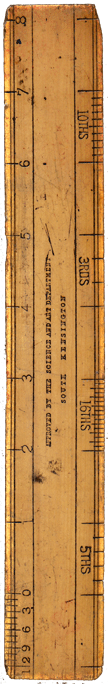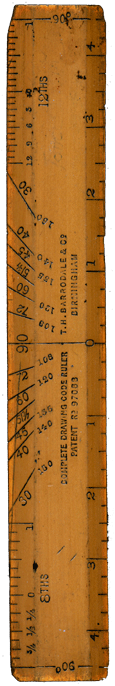# The 10,000-Year-Old Inch

## by Nigel CorriganAlthough the Metric System was devised to simplify calculations it may well represent a major blunder by the Frenchmen responsible. It seems that a brilliantly simple system existed well over 10,000 years ago; one which related not only to the size of the Earth but also to mathematical constants such as π, Pi. In comparison to the arbitrary metre, ancient measures may have been perfectly 'natural', embodying the two most important aspects of any logical system of measurement: 'natural" time and distance. Within this system the fundamental sub- unit, and the one which linked mathematical constants with natural aspects appears to have been the Inch, now relegated to 2·54 centimetres. The Inch: a Natural Unit of Measurement Metrication of measurement was initially proposed as a means of simplifying calculations. The basic unit, the metre, at first sight seems to have many advantages. One metre is l00cm or l000mm. Very simple calculations of addition or subtraction are indeed made easier but the metre has a major weakness: it is an arbitrary length which doesn't relate to any natural measurement. As a consequence there can be no logical relationship between two or more 'natural' functions, such as distance and time, and any relationship to mathematical constants could notpossibly exist. The French, who originated the metre in 1792, decided that any basic unit of measure should, 'naturally' be based on the size of the Earth. Unfortunately the surveyors and mathematicians, rather than using the Equatorial circumference, chose the distance from the Equator to the North Pole. If the Earth were a perfect sphere then the metre would be a natural unit of measure. The Earth is not a sphere: the Equatorial circumference is some forty nautical miles greater than the Polar. The originalmetre wasdefined as one ten-millionth of the distance from Equator to Pole but, as no allowance was made for the flattening at the Poles, this was useless for navigation. As recently as 1960 the metre was re-defined (for the third time), and became 1,650,763·73 wavelengths of the reddish-orange light emitted by the isotope krypton-86 in vacuo. In contrast to this arbitrary measure, the Inch has a natural harmony not only with time and the size of the Earth but also with geometrical constants such as π. The basis of this proposition is another unit of measurement, supposedly taken from the length of an average man's forearm, the Cubit. It is this unit which is accepted as having been used in the construction of the Pyramids. Reference books on the subject agree on one point: the Cubit was 'about' 18·25 inches. It is now suggested that the Cubit was 18·24 inches, precisely.

Evidence to support this is on view in Europe, ironically in Paris. The Obelisk of Luxor, now standing in the Place de Concorde, was presented to the French by the Viceroy of Egypt in 1831. Weighing over two hundred tons, the monument was carved from a single piece of red granite at about the time the Pyramids were constructed. It is reasonable to assume that the stonemasons involved would have used the standard measuring-unit of the time.

Today, we see this obelisk as being 76 feet tall, but how did the stonemasons see it? If the Cubit is indeed 18·24 inches, then the monument was designed to be 76 x 12/18·24, or 50 Cubits precisely. A reasonable figure to select, one might suppose, when the structure was still 'on the drawing-board'. Pyramids were also designed using the standard Cubit. Experts are undecided on the original dimensions of the Great Pyramid of Cheops. We know what they are today, after thousands of years of erosion, but what did the original plans show?

The base of the Pyramid measures 756 feet, or 9,072 inches or 497·3 Cubits. It would be reasonable to consider that the original base-length was 500 Cubits, and that erosion has removed only 2·7 Cubits (less than 17 inches per side). This supports the proposition that the dimensions of the Pyramid are accurately related to the size of the Earth: addition of base dimensions gives 2,000 Cubits or 36,480 inches, which is precisely one-half nautical mile, or 30 seconds of arc at sea level.

Consider the Cubit in relation to the size of the Earth: the (equatorial) circumference is 21,600 nautical miles, or 1,575,936,000 inches (an impractical figure); but what if the unit of measurement was the 18·24-inch Cubit?

Divide 1,575,936,000 inches by 18·24 and we have 86,400,000 Cubits, a number which will immediately be recognized by professional navigators as the number of thousandths of seconds in a 24-hour day. As the Cubit appears to have been defined by the predecessors of today's navigators, this should come as no surprise!

Fortunately for modern-day navigators the sexagesimal, or base-sixty system has survived within their field. 360 degrees, or 21,600 minutes, or 1,296,000 seconds still make up a full circle, as do 60 minutes an hour and 60 seconds a minute. As navigation is simply the relationship of distance, direction and time, this arrangement is perfectly logical; but how can the Cubit relate to both time and distance in easily-managed numbers?

If we accept that the circumference of the Earth is 86,400,000 Cubits, then 1 degree of arc = 240,000 Cubits and l minute = 4,000 Cubits - which is of course the one nautical mile used by navigators today; but as a sub-unit 4,000 Cubits perhaps makes more sense than 6,080 feet* or decimal fractions of the same**.
(* This is the UK Nautical mile, rejected by the metricators. ** 100 fathoms= 1 cable; 10 cables = 1 nautical mile.-Ed.)

In Solar time, 24 hours results in the passage of 86.4 million Cubits on the surface of the Earth below a vertically overhead sun. One hour would see the passage of 3,600,000 Cubits, one minute 60,000 Cubits - and one second, 1,000 Cubits.

### Thus. the apparent passage of the sun over the Earth is precisely 1,000 Cubits per second

What of a mathematical relationship between 18·24 and accepted constants?

A radian is the central angle inscribed in a circle and subtended by an arc equal in length to the radius of the circle. This angle is 57o17'48·8". For most applications 57·3o is used. The related constant, π, was supposedly discovered by the Greeks only 2,000 years ago. However, π can be approximated by dividing one radian by the number of inches in a Cubit: 57·3 divided by 18·24 = 3·141... (and, of course, the number of inches in a Cubit can be found by dividing one radian by π: 57·3/3·141 = 18·24.).

Could 18·24 be as much a numerical constant as π? A definite relationship does exist between 18·24 and the 360o of a complete circle:
360 / (18·24 x 2) = 9·868, whose square root is 3·141, π.
Another:
18·24 x 180 = 3,283, whose square root is 57·3, a radian.

So, was the Cubit an ancient, accurate navigational unit?### Editor's Note

Mr. Corrigan's fascinating speculations continue from this point to develop an equally absorbing possible explanation of the Atlantis legend.